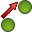# TP Card

With the TP card points (previously defined with the DP card) can be translated, rotated and/or scaled (relative to the origin).

On the Construct tab, in the Modify group, click theTransform point (TP) icon.

## Parameters:

Use label selection
If this option is not checked, then the TP card applies to all the previously defined points. If this option is checked, then a label selective processing is possible.
Move all points starting from label
Together with ending at label specify the label range of points that must be translated, rotated or scaled. See the general discussion of label ranges. If the second field is left empty, only structures with the label set in the first field are considered.
ending at label
Together with Move all points starting from label specify the label range of points that must be translated, rotated or scaled. See the general discussion of label ranges. If the second field is left empty, only structures with the label set in the first field are considered.
Label increment for moved points
Each transformed point will be assigned a label that is the label of the original point incremented by this value. The exception are points with label 0 — their label is not incremented, it remains 0.
Rotation around the X 軸
Angle of rotation ${a}_{x}$ around the X 軸 in degrees.
Rotation around the Y 軸
Angle of rotation ${a}_{y}$ around the Y 軸 in degrees.
Rotation around the Z 軸
Angle of rotation ${a}_{z}$ around the Z 軸 in degrees.
Translation along the X 軸
Translation ${△}_{x}$ in the X direction in m (scaled by the SF card).
Translation along the Y 軸
Translation ${△}_{y}$ in the Y direction in m (scaled by the SF card).
Translation along the Z 軸
Translation ${△}_{z}$ in the Z direction in m (scaled by the SF card).
Scaling in
In this group the user may choose whether scaling is in the X direction, Y direction or Z direction or a combination of these.
Scale factor (after translation)
The scaling factor $\gamma$ , with which the point is scaled after rotation and translation (if the parameter R7 is not specified, it defaults to $\gamma =1$ ).

If a point is rotated around more than one axis with a single card, it is rotated first by an angle ${\alpha }_{z}$ around Z 軸, then by ${\alpha }_{y}$ around the Y 軸 and finally by ${\alpha }_{x}$ around the X 軸. A more detailed description of the transformation can be found in the description of the TG card.

In an exception to the rule that all geometry cards must appear before the EG card, this card (as well as the DP card) can be used after the EG to define points for use in the AP card.